## Introduction

An important question in evolution, ecology and astrobiology is: what fundamental limitations are present for the simplest organisms and what limitations might have been present when life emerged and started to evolve? It is important to understand how limitations differ for broad classes of organisms as this informs competitive dynamics and motivates evolutionary trajectories and transitions. Although speciation is the subtle process of unique evolutionary trajectories, it should be noted that these trajectories occur within boundaries defined by basic physical, energetic and chemical limitations. For example, scaling laws relating numerous organism features to body size often illustrate a shared physical limitation that, on the average, organizes biological features (e.g., Niklas, 2004; Brown et al., 2004; West and Brown, 2005; DeLong et al., 2010). Recent work has shown that these scaling laws can be connected with metabolic partitioning to anticipate the smallest possible bacterium (Kempes et al., 2012). Indeed, in the fields of bioengineering, ecology and evolution, there are a large number of known scaling relationships, and mapping the entire space of physical, energetic and chemical limitations is a significant task of central consequence for evolution and ecology (Brown et al., 2004).

Here we present an analysis, which we verify using compiled data, of the changes in molecular, physiological and structural composition across the entire range of bacterial cell sizes. Specifically, we examine the total amount of the following components: DNA, ribosomes, proteins, cell membrane, tRNA and mRNA. For each of these features we often focus on power-law relationships for various organism features, and when two properties ‘scale’ with different exponents, this often implies the onset of a limitation at a given size which may have important evolutionary consequences. Using this framework we show that the interconnection between energetic, physical, informational (genomic), chemical and temporal processes leads to predictions of the upper and lower boundaries of bacterial size and defines the evolutionary flexibility for bacteria between these two bounds. This perspective, which spans a huge diversity of species and five orders of magnitude in cell volume, adds explicit cross-species and size-based dependencies to the large body of work on the interconnection between various cellular processes (e.g., Tempest and Hunter, 1965; Tempest et al., 1965; Simon and Azam, 1989; Bremer et al., 1996; Cayley and Record, 2003; Dethlefsen and Schmidt, 2007; Zaslaver et al., 2009; DeLong et al., 2010; Scott et al., 2010; Goehring and Hyman, 2012; Kempes et al., 2012; Turner et al., 2012; Lloyd, 2013; Burnap, 2015). Furthermore, our work connects these cross-species trends to previous considerations of space limitation for the smallest organisms (Knoll et al., 1999) and the recent observations of small environmental bacteria (e.g., Luef et al., 2015). Knowing these connections may help us to infer ecological trait tradeoffs, quantify the constraints in designing organisms or interpret long-standing environmental observations such as the Redfield ratio for nutrient proportionalities in the ocean (Geider and La Roche, 2002).

Finally, most of the cellular components analyzed here should have a deep connection with overall cellular metabolism. In some cases, such as the processes determined by growth rate (Kempes et al., 2012), metabolic rate explicitly constrains component requirements. However, we find that it is more difficult to determine what cellular features set overall metabolic rate, which scales superlinearly for bacteria in contrast to other classes of organisms (DeLong et al., 2010). In fact, we find that there is no obvious connection between the scaling of any single cellular feature and the overall metabolic rate, highlighting the continuing mystery of metabolic scaling in bacteria. We also find increasing trends in the overall efficiency of bacteria defined as the metabolic power per genetic and protein content, which is surprising given previous considerations (Lane and Martin, 2010).

## Materials and methods

Our overarching goal is to understand how the requirement for each cellular component depends on total cell size so that we can constrain the limits facing cells at various scales. We approach these constraints from both energetic perspectives and overall space constraints, the latter of which often derives from energetics via the requirements of certain rate processes (e.g., growth rates; Kempes et al., 2012). For the space constraints our analysis focuses on both the scaling of individual components and the sum total of all cellular components. Thus, it is useful to define the total volume of the cell, Vc, as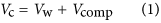where Vw is the total volume of water in the cell and Vcomp is the volume of cellular components defined as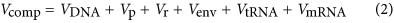where VDNA is the total volume of DNA in the cell, Vp is the protein volume, Vr is the ribosomal volume, Venv is the volume of the cellular envelope, VtRNA is the volume of tRNA and VmRNA is the total mRNA volume. One of our main efforts here is to determine the sizes at which single components or the total component volume limits cell size, and the combination of the both types of analysis allows us to infer tradeoffs across the range of bacteria. Additionally, it is useful within our considerations to define the volumetric fraction, fd, of the cell that is dry weight as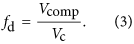In general, we describe the scaling of a generic cell component (e.g., DNA) as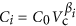, where βi is the exponent for volume (measured in m3 throughout this paper) dependence of the component. It is important to note that if βi<1 then component Ci will be a decreasing fraction of total cell volume with increasing cell size, while βi>1 leads to an increasing fraction of cell volume. In either case, if βi≠1, then there will be a size at which the component volume equals total cell volume, implying a limitation because extrapolation beyond this point would produce Ci>Vc, which is impossible. It should also be noted that if some of the terms in Vcomp scale with βi≠1 then other terms cannot be simple power laws in order for the equalities in Equations (1) and (2) to hold. We will see that several aspects of the cell do not follow simple power-law relationships with overall cell volume. It should be noted, more generally, that if any component depends on Vc in a manner that is not a simple proportionality then the fractional composition of the cell will change across cell sizes, and many nonlinear functions will lead to critical sizes beyond which the volume of a component will exceed Vc, a physical impossibility that implies a constraint on size.

The basic perspective of physiological scaling is supported by many previous observations of organism features that follow power-law relationships; however, it should be noted that in many of the cases studied here more complicated relationships are warranted as illustrated, for example, by previous efforts on growth rates (Kempes et al., 2012). Furthermore, many cellular features are expected to be connected mechanistically with others (e.g., growth rates and ribosome content), and Figure 1 illustrates these interconnections, along with the cascading dependence of many cellular features on total cell volume. We begin with the scaling of cellular energetics given the central role that these will play in our analysis and the previous demonstrations that energetics can be used to derive other features such as growth rate (Kempes et al., 2012).

### Metabolic rate

Previous work has shown that metabolic rate, B (W), scales with body size according to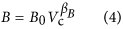where βB≈2 in bacteria (DeLong et al., 2010) (βB≈1.7 for an OLS fit) and B0 is a normalization constant with units W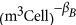. Although most previous allometric studies focus on mass as the size unit for organisms, we use cell volume, Vc, here because this is typically what is directly measured in bacteria and, as can be inferred from our later findings, conversion to mass units can be complicated as cell density is not constant with cell size (we later find that density is greatest for the smallest and largest bacteria with a minimum value of 1.06 × 106 g m−3 for an intermediate cell size of 4.92 × 10−18 m3; see Supplementary Figure S5). Furthermore, many previous studies report wet masses, which were the result of applying a constant density factor to measured volumes, so conversion to volume units is straightforward: Vc=mw/dc, where dc=1.1 × 106 g m−3 is the previously used cell density assumed to be constant and mw is the calculated wet mass from previous studies (e.g., West and Brown, 2005; Makarieva et al., 2008; DeLong et al., 2010). It should be noted that this conversion will not change the scaling exponents found in studies that use wet mass provided that these studies used a constant value for dc.

### Growth rates

To derive growth rates, it has been demonstrated (Kempes et al., 2012) that the metabolic scaling relationship from the preceding section can be combined with the concept of metabolic partitioning according to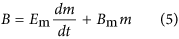where Bm (W g−1) is the unit maintenance metabolism and Em (J g−1) is the unit cost of biosynthesis. Both of these parameters can be derived from bulk community energetic constants of the yield (e.g., g cells per mol resource) and maintenance (mols of resource consumed for survival alone) coefficients, measured, for example, in a chemostat and converted to energy units (e.g., J per mol of resource; see Kempes et al., 2012 for a more detailed presentation). The partitioned metabolism in Equation (5) has been used to derive a single-cell growth curve (Kempes et al., 2012), from which it is possible to determine the generation or division time, td (s), of a single cell, along with the cross-species population growth rate, μ≡ln(2)/td (s−1), of bacteria following the relationship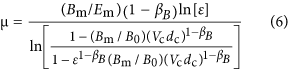where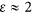is the ratio of the size of the cell at division compared with its initial size. Our efforts here largely concern the physiological requirements of a single cell, and thus most of our derivations (e.g., Supplementary Equations (S6)–(S47)) rely fundamentally on the division time td; however, the final results appear as functions of ln(2)/td and so it is convenient to report equations in terms of μ. It should also be noted that we have presented this equation with the previous conversion (Kempes et al., 2012) between cell volume and wet mass explicitly stated, where dc (g m−3) is the cell density. The relationship for μ in Equation (6) demonstrates an asymptotic behavior for the smallest bacteria that limits the smallest possible cells (Kempes et al., 2012) and approximates the power law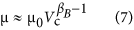for larger cell volumes (see Supplementary Information) showing that growth rate increases rapidly as bacteria become larger. It should be noted that in this framework Vc is the only true variable while all of the other parameters are, on average, expected to be constants, which has been supported by data (Kempes et al., 2012). However, it should be noted that the model could incorporate future discoveries of unknown dependencies of any of the constants; for example, Bm could have a complex response under extreme energy limitation, which is a regime that has not yet been well characterized. The overall growth rate, as represented by μ, defines the dominant rate processes of the cell, and we will later show that this connection is central to determining the number of ribosomes, tRNA and mRNA.

Given the strong dependence of growth rate on cell size, we should furthermore expect significant shifts in macromolecular content for bacteria of different volumes. This is consistent with previous work showing that the growth rate of bacteria is strongly connected with the relative abundance of various macromolecular components, most notably ribosomes, RNA and proteins (e.g., Bremer et al., 1996; Zaslaver et al., 2009; Scott et al., 2010; Burnap, 2015).

### Genome size

It is interesting then to consider how the genomic complexity of bacteria changes in comparison with the patterns in metabolic and growth rates. As bacteria are becoming larger how rapidly is their genome increasing? Previous efforts have shown that genome size has a strong scaling with cell size in bacteria (Shuter et al., 1983; West and Brown, 2005; DeLong et al., 2010) and here we consider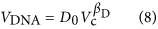where we later determine D0 and βD from a compilation of data and previous compilations.

### Protein scaling

The genomic scaling law is expected to be a powerful tool for understanding basic constraints on bacteria because it should influence many other features of the cell. For example, the total volume of proteins can be related to genome length by knowing how many proteins are produced per gene:where(m3) is the volume of an average protein,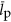(bp) is the average length, in nucleotides, of a protein encoding gene and(copies cell−1) is the average copy number of a protein, and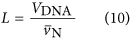is the length of the genome given the average volume of a nucleotide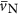(m3) (see Supporting Information and Supplementary Figure S1) which allows us to write the volume-dependent form: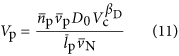In Equation (11),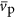,and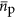could each scale with cell volume. This relationship for protein volume specifies the explicit relationship with protein copy number and the scaling of genome size with cell volume, however, for simplicity in presenting results we consider and report the analogous scaling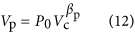which can be easily connected with Equation (11).

### Model of ribosome requirement

We derive the ribosome requirement by considering how many ribosomes are required to replicate all ribosomes and proteins within a division cycle while also replacing proteins and ribosomes that have been degraded. This perspective is complicated by the fact that as ribosomes and proteins are dynamically produced they contribute, respectively, to the biosynthetic capacity or biosynthetic requirements of a cell. This leads to a conceptually simple, but algebraically complicated derivation, which we present in the Supplementary Information, with the simple final ribosome requirement that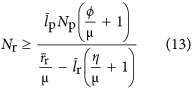where lr is the average length of a ribosome in base pairs, Nr is the number or ribosomes in the cell, rr (bp s−1) is the maximum base pair processing rate of the ribosome which is assumed to be constant across both taxa and cell size, η (s−1) and φ (s−1) are specific degradation rates for ribosomes and proteins, respectively, and Np is the total number of proteins given by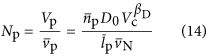which depends on overall cell volume. The total number of proteins could also be found using Equation (12) as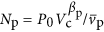when P0 and βp are known. In Equation (13) it is important to note that μ is a function determined solely by overall cell size given that the other parameters in Equation (6) are expected to be pure constants. In the Supplementary Information we present Nr with the dependence on Vc, via μ and Np, explicitly stated (Supplementary Equations (S46) and (S47)). Our relationship for the number of ribosomes can be converted to the total volume of required ribosomes in the cell: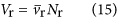where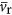is the average volume of a ribosome (see Supplementary Information for the explicit dependence on Vc).

An alternative perspective, commensurate with our considerations of the other cellular components, is that the number of ribosomes follows a simple scaling with cell volume: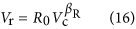and we also test this possibility when analyzing trends in the quantity of ribosomes.

### Trends in other RNA

In relationship to the number of ribosomes we must also consider the requirements for tRNA and mRNA. The minimum number of tRNA and mRNA in the cell should be equal to the number of ribosomes so that every ribosome is engaged in protein synthesis and the cell is maximizing the biosynthetic rate. However, this does not consider the time scales required for mRNA and tRNA to find and ‘react’ with the ribosome. Another reasonable assumption is that there is some local concentration of tRNA and mRNA which allows the ribosome to operate at its maximum capacity. If this concentration is held constant, then the total number of tRNA and mRNA in the cell should be proportional to the number of ribosomes, as should the total volume of each of these components. Thus, we have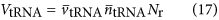whereis the average volume of a tRNA, and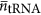is the average number of tRNA per ribosome, andwhere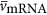is the average volume of an mRNA and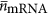is the average number of mRNA per ribosome. It should be noted that Equations (17) and (18) both depend on cell volume because Nr is a function of Vc, and the explicit form is included in the Supplementary Information. Our estimates for the number of mRNA per ribosome are in fact close to one, implying that most ribosomes are engaged in protein synthesis (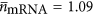, see Supplementary Information).

### Cellular envelope

Completing our analysis of cellular composition we consider the volume of the cellular envelope which we can approximate, considering a spherical cell, as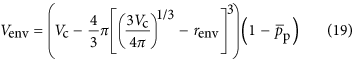where renv (m3) is the effective thickness of the cellular envelope and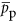is the average percentage of the envelope that is occupied by proteins (we find that this is roughly equal to 0.15, see Supplementary Information). It should be noted that renv will vary depending on whether the cell is Gram negative or positive.

### Predicting limiting behavior

A key aspect of our scaling analysis is that scaling exponents that deviate from 1 imply that there is a distinct bounding size at which a given component will equal and then exceed the total cell volume. This represents an inferred lower bound for β<1 and an upper bound for β>1. For example, the relationship for genome volume (Equation (8)) gives the size of a cell that is entirely filled by DNA, VDNA=Vc, as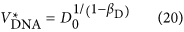and, from Equation (12), the volume at which proteins are predicted to entirely fill the cell would be given by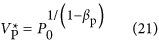Similarly, a major prediction of the ribosome requirements (Equation (13)) is the appearance of two asymptotes at both the small and large end of bacterial sizes. The small-end asymptote is characterized by the previous observation that the division rate will become zero for a cell volume of roughly 1.45 × 10−20 m3 (Kempes et al., 2012). The large-end asymptote is characterized by the point at which the time to divide is not sufficient to replicate just the ribosomes, and in the context of Equation (13) this is the point when the denominator is less than or equal to zero leading to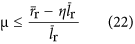which we term the ‘ribosome catastrophe’.

Beyond these single component limits we also consider the limitation faced by the combination of all overall cellular components given in Equation (2). Here it should be noted that some of the subcomponents follow power laws, others do not (e.g., RNA components), and the overall relationship, which represents a sum, has a more complicated nonlinear form than a simple power law. However, it is still possible to solve for the point at which Vtot=Vc, where it should be noted that the errors associated with each scaling may become amplified at the largest and smallest scales. In plotting the relationships, either predicted or fit, for single components and the total component volume we extrapolate the fits to regions where these volumes would exceed the total cell volume. This is physically impossible, but we make these extrapolations to show the scale of the challenge faced at certain sizes in terms of component requirements.

## Results and discussion

### Basic scaling relationships and component trends

Figure 2a gives our own compilation (which includes data from Shuter et al., 1983; West and Brown, 2005; DeLong et al., 2010) of genome size, VDNA, showing that the scaling follows Equation (8) with D0=3.0 × 10−17 (m3 DNA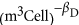) and βD=0.21±0.03 (see Supplementary Information for the 95% confidence intervals on all reported normalizations constants such as D0). As previously noted (DeLong et al., 2010), this scaling shows that genome size is increasing much less steeply than metabolic rate, which is perhaps surprising, although it is not clear how the number of genes should explicitly determine overall metabolism. For example, perhaps metabolic rate should be related instead to the number of copies of certain pathway genes or to the concentration of specific proteins in the cell (Burnap, 2015).

Our analysis, which includes data compiled in Tempest and Hunter (1965), Tempest et al. (1965),Simon and Azam (1989), Dethlefsen and Schmidt (2007), Milo (2013) and Valgepea et al. (2013), shows that the scaling for the volume of protein compared with that of overall cell volume is also less than linear, with P0=3.42 × 10−7 (m3Protein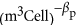), and βp=0.70±0.06 (Figure 2b). Considering the observed scaling for protein volume and DNA volume in the context of Equation (11), we calculate that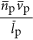scales like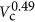. It is likely thatandare roughly constant across cells (e.g., the average gene length has been found to be approximately invariant across all bacteria (Xu et al., 2006)) and, therefore, this result implies that the average copy number of proteins is likely increasing as cells become larger. Thus, not only are the number of unique proteins increasing for larger cells and genomes but the number of times that each protein is copied is also increasing.

The departure of protein scaling from genome scaling gives an example of a cellular feature that is not simply predicted by a proportionality with overall genome size (e.g., the surprising scaling of VpL3.33 given βp/βD≈3.33). Given that total protein content is expected to play a strong role in determining the number of ribosomes (Equation (13)) and the overall RNA content of the cell, we should also expect these features to have a complicated connection with the genomic complexity of a bacterium.

We predict the relationship between the total volume of ribosomes and overall cell volume from Equations (13), (14),(15) using the asymptotic form of μ (see Supplementary Information for explicit forms) and measured values for the degradation rates φ and η. This prediction should represent a true lower bound, and in Figure 2c this curve (dashed line) tracks, but is consistently smaller than the observed volume of ribosomes from our cross-species compilation of published data, which includes Bremer et al. (1996), Fegatella et al. (1998), Seybert et al. (2006) and Luef et al. (2015). We also find a relationship for the number of ribosomes using a best fit of the degradation rates with the power-law approximation of μ (see Supplementary Information), which accurately captures the cross-species trends in ribosome volume in Figure 2c. We find that this fitted prediction, which relies on only a single free parameter, has a very similar goodness of fit to a simple power law, where we find that the best fit to Equation (16) is given by R0=1.54 × 10−7 (m3Ribosomes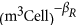) and βR=0.73±0.15. It should be noted that the best fit of our full model for ribosome composition makes predictions for ranges of cell size where we do not have data to test the model. Some of our later conclusions and inferences rely on these predictions, and thus provide a set of testable and open hypotheses, such as the number of ribosomes found at larger bacterial sizes or the exact size at which the ‘ribosome catastrophe’ occurs.

### Shifts in cellular composition and the smallest bacteria

Our analysis now provides the size dependence for the major cellular components across the domain of bacteria. This includes the prediction for the volume of the envelope considering three cases: only a single membrane (organisms without a cell wall) and the effective thickness for Gram-negative or -positive bacteria (Figure 2d), and predictions for tRNA and mRNA given the volume of ribosomes.

Of critical interest is how the relative cellular composition changes across species of diverse size and what tradeoffs and limitations we might be able to infer from these shifts. Figure 3a shows all of the cross-species trends for various cellular components compared with the overall cell volume. The compiled trends show that the composition of the cell greatly shifts across the range of bacterial body sizes. The smallest cell volumes are dominated by DNA and, to a lesser extent, cell membrane and protein, while the largest bacteria are composed of mostly ribosomes, tRNA and mRNA. These changes in composition are accompanied by shifts in the dry fraction of cells, as discussed later, and the overall cell density (see Supplementary Figure S5 and related discussion).

These trends also predict at what scale certain components would become limiting. For example, the relationship for genome volume gives the mass of a cell that is entirely filled by DNA as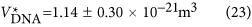which is expectedly smaller than the smallest observed organism by a factor of approximately three (Table 1). This result shows that for the smallest cells there is not much room beyond the basic requirements for the genome. Similarly, the volume at which proteins are predicted to entirely fill the cell would be given by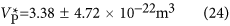which is about an order of magnitude smaller than the smallest cell. The cellular envelope volume that completely fills the cell can be found using Equation (19) given values for the envelope thickness. For a Gram-negative bacterium, the envelope would fully occupy the cell at a size of 7.44 × 10−22 m3, for a Gram-positive, at a size of 2.91 × 10−23 m3, and for a single membrane (organisms lacking a cell wall), at a size of 1.01 × 10−25 m3. These results show that for the smallest cells the envelope represents a significant portion of the overall volume. In order to reduce the required envelope volume, the smallest cells are expected to minimize surface area by becoming increasingly spherical, which agrees well with the mostly spherical cell shapes reported by Luef et al. (2015), and are expected to reduce envelope thickness or layers, consistent with Mycoplasma that lack a cell wall.

The above analysis is useful for understanding which individual components become limiting at the smallest cell sizes. More generally, we can understand the overall space constraints of the cell at the small end of life by considering the total volume from Equation (2). This summed component volume will equal the total cellular volume for a size of 1.02 × 10−20 m3 for a Gram-positive bacterium, which is comparable to the previous energetic prediction (Kempes et al., 2012; Figure 3b) and larger than the smallest observed and hypothesized cells by roughly a factor of two (Knoll et al., 1999; Seybert et al., 2006; Luef et al., 2015). Considering only a single membrane would give a minimal size of 4.10 × 10−21 m3, which closely matches measurements for the smallest bacteria (Table 1). Considerations of the space for the smallest cells is a topic that has been deeply considered in the past and previous estimates agree with our prediction for a lower bound, where it was estimated that the minimum size required for a cell of modern biochemical complexity should fall in the range of 4.19 × 10−21–1.41 × 10−20 m3 (Knoll et al., 1999). Furthermore, this result, and our space constraint estimate from Vcomp=Vtot, also compare well with the limit anticipated from energetic considerations (Kempes et al., 2012), thus highlighting that multiple constraints are likely limiting the possibility of becoming smaller at the smallest scale of life.

Our result shows that the average cross-species trends converge to predict the smallest cell at the appropriate size range while still being an accurate predictor of cellular composition as cells move orders of magnitude away from this lower bound (Figures 2 and 3). This is remarkable because our analysis shows that average trends in cellular composition across the diversity of bacteria are consistent with the scale of the lower limits of life. However, these predictions also highlight necessary tradeoffs for the smallest cells, where the total volume requirements accurately predict the observed minimum size only for a reduced cellular envelope of a single membrane. This may not be biologically feasible and thus other compositional tradeoffs may be required for the smallest cells. For example, the genome size for Mycoplasma genitalium is 5.8 × 105 bp (Moya et al., 2009), which is about half the size of the prediction from the average trend for a bacterium of this volume. Similarly, at the smallest sizes, it is possible that cells could evolve to have lower copy number of proteins per gene, or have smaller average protein sizes—all to accommodate cell volume constraints.

Our own previous cross-species considerations (Kempes et al., 2012) predict a smallest bacterium that compares well with the mean cell size of Luef et al. (2015) and Seybert et al. (2006) but is about four to five times larger than the smallest cells from these studies (Table 1). This difference highlights that the smallest organisms may deviate from average energetic properties, such as unit maintenance costs, in addition to compositional shifts, though some of these deviations would require a cellular biochemistry that differs from modern examples. For example, previous estimates for possible primitive cells would lead to a size of 3.59 × 10−24 m3 (Knoll et al., 1999), which is not much bigger than the size at which the cell volume is entirely filled by a single membrane (1.01 × 10−25 m3).

Although the data presented here are for the domain of bacteria, we may find similar constraints in archaea. For example, the smallest observed archaea are roughly comparable to the smallest bacteria in volume (≈3.41 × 10−20 m3; Huber et al., 2002; Comolli et al., 2009) and have similar genome sizes (0.5 megabases (Huber et al., 2002)) and ribosome counts (≈92; Comolli et al., 2009) at this size.

### RNA limitations and the largest bacteria

For the largest bacteria, the dominant cell components are determined by the increasing need for processes related to transcription and translation to match increasing growth rates. As discussed earlier, this eventually leads to a ‘ribosome catastrophe’, where a finite size would require an infinite number of ribosomes, tRNA and mRNA. The limits anticipated in our model for the volume of ribosomes can be seen in the prediction curve of Figure 2c, where the entire cell volume would be filled by ribosomes, the ‘ribosome catastrophe’, at a size of 1.39±0.03 × 10−15m3. This is in contrast to the smallest bacteria, where the need for ribosomes, tRNA and mRNA are predicted to diminish based on the decreasing growth rates. Previous predictions predict that growth rate goes to zero at a size of 1.45 × 10−20 m3 (Kempes et al., 2012).

Expanding from the number of ribosomes to the total RNA content, the prediction from Equation (18) compares well with measurements for mRNA in Escherichia coli and Mycoplasma pneumoniae (see Supplementary Information for the value of the constants in Equations (17) and (18)). For E. coli, previous measurements give a range of 1380 (Neidhardt et al., 1996) to ≈10 000 mRNA per cell (see compilation in the Supplementary Information of Lu et al., 2007), compared with our estimate of 2540 to 12 257 using a cell volume between roughly 0.7 and 6 μm3 (Dethlefsen and Schmidt, 2007; Milo, 2013), and dividing Equation (18) by. For M. pneumoniae, the mRNA quantities are two orders of magnitude lower than E. coli at around 10 per cell (Maier et al., 2011), which is similar to the lower bound of our estimate of 68-1159 given the considerable range in observed cell size of 0.005–0.24 μm3 (Seybert et al., 2006).

Similarly, the number of tRNA per cell in E. coli has been observed to range between 52 000 and 375 000 (Jakubowski and Goldman, 1984; Neidhardt et al., 1996; Mackie, 2013), where we would predict a range of 23 561 to 113 707 based on the range of cell volumes used above. For M. pneumoniae, observations give 190 tRNA per cell (Maier et al., 2011), which is similar to our prediction of 631 to 10 755. It should be noted that in both cases we overestimate the number of tRNA and mRNA in Mycoplasma which is likely due to the considerable space constraints faced by these minimal organisms, as discussed earlier.

Parallel to our analysis of the smallest cells, the total component volume also sets an upper bound on size, where Vcomp=Vtot at a size of 1.19 × 10−15 m3. This limit is dominated by the ‘ribosome catastrophe’, where the cell will require more ribosomes than can fit in its volume in order for biosynthesis to keep up with increasing growth rate. It should be noted that this predicted upper bound is four orders of magnitude smaller than the largest observed bacterium of ≈4.19 × 10−12 m3 (Schulz et al., 1999). However, this giant sulfur bacterium (Thiomargarita namibiensis) is dominated by vacuoles used for nutrient storage, which does not represent the metabolic active or relevant volume considered in our model of the required components (Schulz et al., 1999). If we remove the vacuole volume (approximately 98% of the total cell volume; Schulz et al., 1999) then we are left with a size of 1.05 × 10−14 m3, which is only an order of magnitude larger than our predicted upper bound. It is interesting to consider what tradeoffs these cells have made to avoid the ‘ribosome catastrophe’, and one possibility is that the environment regulates growth at a slower rate than cell size would dictate.

Similar to our biosynthetic limit, previous work has shown that there is a linear increase in the fraction of promoter activity devoted to ribosomes with increasing growth rate (Zaslaver et al., 2009). If the observed trend is extrapolated, then at a growth rate of 2.9 divisions per hour all promoter activity would be devoted to ribosomes, which is not feasible, and this would correspond to a cell volume of 2.1 × 10−17 m3. This predicted upper bound is smaller than our space limitation and highlights the multiple constraints that could be faced for the largest bacteria. Both of these upper bounds on cell volume correspond to the rough size at which there is an observed transition to eukaryotic life, along with corresponding and dramatic shifts in growth and metabolic rates (DeLong et al., 2010; Kempes et al., 2012). Thus, this major transition may in part be motivated by the challenges related to biosynthesis and ribosome requirements.

### Free volume

In between this lower and upper limit, changes in composition can be most easily understood by considering the total physical space required for essential components compared with the remaining volume. Considering the average composition trends that we have discussed thus far, the fraction of the cell that is dry weight (Equation (3)) is 1 for the smallest bacteria and decreases quickly for larger cells until reaching a minimum around 0.13 at a size of 2.83 × 10−17 m3, before increasing again towards the largest cell (Figure 3c). From this relationship we can see that bacteria of intermediate size have the most free volume, and the maximum of this volume occurs around the size of many well-studied species such as E. coli.

This free volume, which is an inferred and testable prediction from our model, has important evolutionary and ecological consequences because it implies that there is a range of cell sizes that may have the most flexibility due to the lack of physical space constraints. This flexibility could manifest as an increase in the copies of specific proteins in stressful conditions, greater ability to buffer waste products or plasticity in increasing the number of ribosomes for faster growth, among many other possible benefits. This is in sharp contrast to the smallest cells that barely have enough space for basic biochemical requirements. An important avenue of future research is to examine whether and how individual taxa allocate ‘free volume’ differently to various components or functions, and whether such allocation can be understood as an adaptation to specific niches or conditions.

However, it is also important to consider, as part of this ‘free volume’, the volume of water necessary for cellular reactions and internal transport. For example, if all of the free volume were dedicated to water, and given the size of E. coli, we would predict that 67–76% of the cell is filled with water, in good agreement with previous estimates of 70% (Neidhardt et al., 1996). This comparison implies that all of the free volume could reasonably be occupied by water, and that evolutionary flexibility at these cell sizes could depend on the degree to which the water content is constrained. Our predictions suggest that the smallest and largest cells have lower water content, which is consistent with previous efforts that describe a cytoplasm with high molecular packing densities (e.g., von Hippel and Berg, 1989; Cayley and Record, 2003; Golding and Cox, 2006; Burnap, 2015). Similarly, these changes in water content may alter processes related to the solvent capacity or diffusive, sub-diffusive or active transport in the cell (e.g., von Hippel and Berg, 1989; Cayley and Record, 2003; Errington, 2003; Golding and Cox, 2006; Burnap, 2015), and it is of potential future interest to understand whether there are specific and calculable requirements for water content in cells of different size in connection with cellular composition. Furthermore, these results imply that the application of a constant dry weight ratio to diverse species may often be inaccurate.

### Energetic limitations and complexities

Previous studies have highlighted that prokaryotes have a superlinear scaling between metabolic rate and body size. This observation has been successfully used to derive cross-species trends in growth rates and a limit where growth rate should go to zero in bacteria (Kempes et al., 2012). However, the superlinear scaling of metabolism is still not fundamentally understood and here we are surprised to find that every cellular feature scales sublinearly with overall cell volume. This implies that metabolism is not a simple proportionality of any one cellular feature. Figure 4b gives the scaling of the total metabolic rate as a function of the volume of each cellular component where it can be seen that all of the scalings are superlinear. Taken together these observations suggest that metabolic rate is a complicated emergent property of some measure of increasing cellular complexity. In particular, the scaling of metabolic rate with genome size stands out with an exponent close to 8, and it may be the case that metabolism is a complicated function of the growing complexity of the metabolic network which adds capacity quickly with the addition of novel proteins (DeLong et al., 2010). Metabolism also goes up steeply (exponents close to 2.4) with the total envelope volume and total number of proteins which also makes sense given that the membrane surface area controls ATP synthesis (Lane and Martin, 2010) and the number of proteins are the actual components of the metabolic network. The scaling of proteins scales closely to a power-law approximation of the growth rate equation (6) (an exponent of 0.65 compared with 0.70). Since growth rate is set by the overall scaling of metabolic rate (Kempes et al., 2012), it is possible that the protein concentration could be the dominant factor controlling both metabolic rate and growth rate, albeit not via any direct proportionality. Yet the explicit connection between any of these components to the scaling of metabolic rate remains unclear.